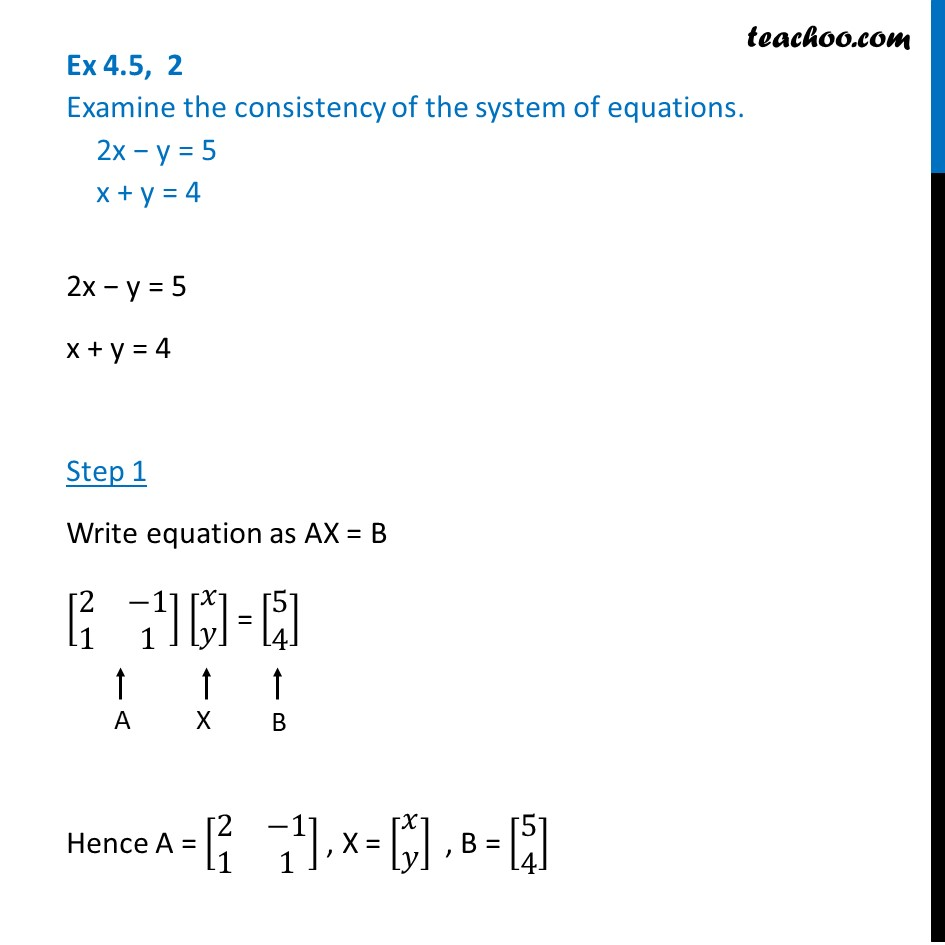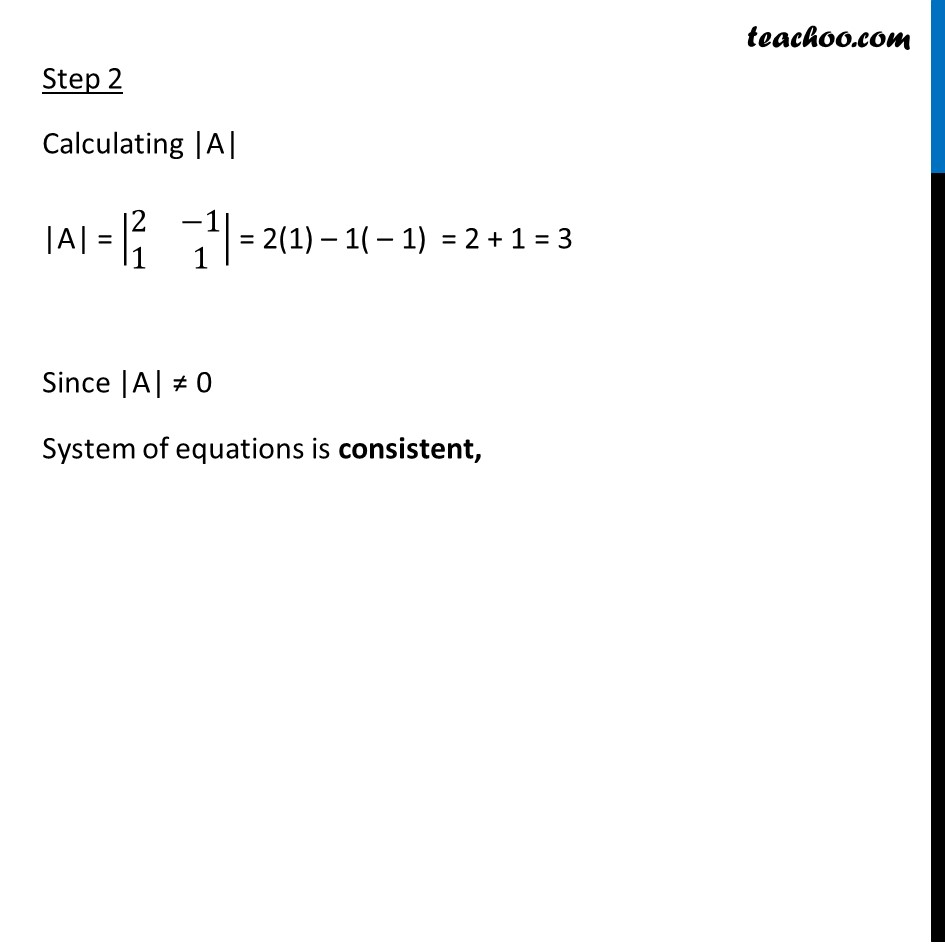Ex 4.5

Chapter 4 Class 12 Determinants
Serial order wiseLearn in your speed, with individual attention - Teachoo Maths 1-on-1 Class

### Transcript

Ex 4.5, 2 Examine the consistency of the system of equations. 2x − y = 5 x + y = 4 2x − y = 5 x + y = 4 Step 1 Write equation as AX = B [■8(2&−1@1&1)] [■8(𝑥@𝑦)] = [■8(5@4)] Hence A = [■8(2&−1@1&1)] , X = [■8(𝑥@𝑦)] , B = [■8(5@4)] Step 2 Calculating |A| |A| = |■8(2&−1@1&1)| = 2(1) – 1( – 1) = 2 + 1 = 3 Since |A| ≠ 0 System of equations is consistent,